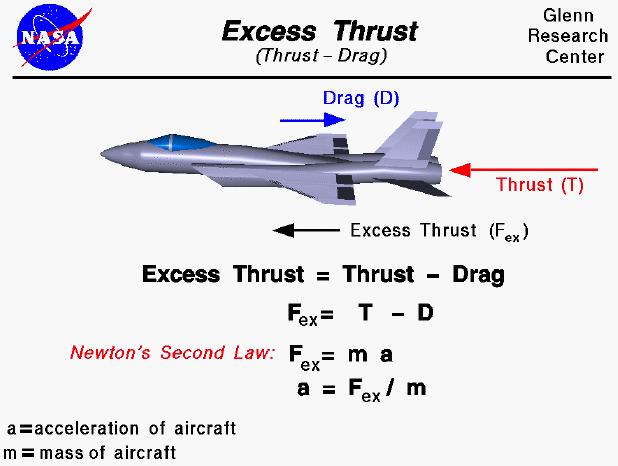The propulsion system of an aircraft must perform two important roles:

• During cruise, the engine must provide enough thrust, to balance the aircraft drag while using as little fuel as possible.
• During takeoff and maneuvers, the engine must provide additional thrust to accelerate the aircraft.

The thrust minus the drag of the aircraft is called the excess thrust . Considering Newton's second law of motion, mass (m) times acceleration (a) is equal to the net external force (Fex). For an aircraft, the horizontal net external force is the excess thrust. Therefore, the acceleration of an aircraft is equal to the excess thrust divided by the mass of the aircraft. The thrust divided by the mass of the aircraft is closely related to the thrust to weight ratio. Airplanes with high excess thrust, like fighter planes, can accelerate faster than aircraft with low excess thrust.

If the excess thrust and the mass remain constant, the basic equation of motion can be easily solved for the velocity and displacement as a function of time. This equation can be used only if the force (and the acceleration) are constant. Unfortunately for aircraft, drag is a function of the square of the velocity. So we can assume a constant force for only a very small amount of time. To solve the actual equations of motion for an aircraft, we must use calculus and integrate the equations of motion, either analytically or numerically.

Guided Tours